I.F.2. The Derivatives of Simple Exponential and Trigonometric Functions  (trig omitted here)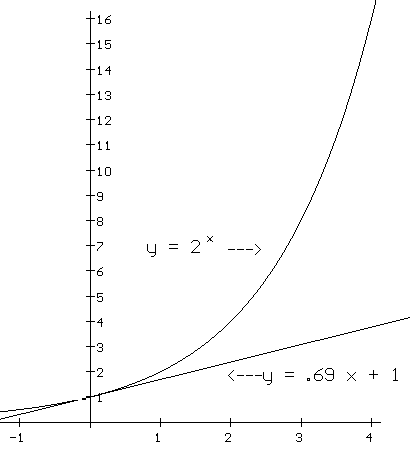Exponential and trigonometric functions play an important role in many models. Simple models for population growth and the cooling of liquids involve careful analysis based on rates of change. These rates force the models to use exponential functions. Slightly more involved models for competing populations and the motion of a spring give rise to trigonometric functions. We will study this kind of modelling later in Chapter VI as we investigate models determined by differential equations.

 h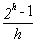1 1 0.01 0.69555501 1e-04 0.6931712 1e-06 0.69314742 1e-08 0.69314718 1e-10 0.69314776
The Derivatives of Simple Exponential Functions. We begin with simple exponential functions. At this stage of our studies we will presume the variables in many models are controlled by an exponential function like f(x) = 2x. In your prior work in Chapter I.A and I.B exercises with tangent lines and rates you may have already explored the derivative of simple exponential functions. We will continue in this section to describe the derivatives of simple exponential functions more completely.

Let's start with f(x) = 2x and estimate f '(0). Looking at the graph of this function in Figure *** certainly supports the belief that f has a tangent line and thus that f should have a derivative at 0. Consider the data in Table ***. It suggests that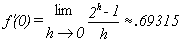.

 x f(x)=2x estimate of f '(x) Estimate of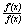-4 0.0625 0.043322 0.6931495 -3 0.125 0.086644 0.6931495 -2 0.25 0.173287 0.6931495 -1 0.5 0.346575 0.6931495 0 1 0.69315 0.6931496 1 2 1.386299 0.6931496 2 4 2.772598 0.6931496 3 8 5.545197 0.6931496 4 16 11.09039 0.6931496
Leaving this issue for a moment we turn to the derivative of f at a, i.e., f '(a). Here it is to our advantage to use the characterization of the derivative as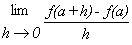. This leads us to examine the difference quotient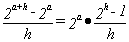. Now as we consider the limit as h®0 we can use f '(0). The fact is that by the definition of the derivative as h®0,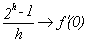. So, to describe f '(a) in general, we see that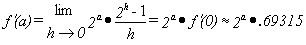.

Remarks: 1. This result does seem quite different from any we have had before because the derivative here is a constant multiple of the value of the function. In other words the derivative is proportional to the value of the function. We can check this is several ways to confirm the truth of the result.

First we can look at the ratio of a numerical estimate of the derivative at several points and see that even at the estimation level the ratio is constant. This is done in Figure *** using h=.0001 for the estimation. Another way to check the result is to draw lines using the function values times .693 for the slope at various points on the graph to see that these lines do appear to be tangent. Of course both these techniques do not fully justify the claim as does the analysis, but they do add to the credibility in making them sensible based on a variety of experiences.

Finally with technology you might match the graph of a numerical estimation of the derivative against the graph of .693 times 2x and notice how close these graphs appear.

2. The general exponential function with a base b ( not equal to 1) can be treated in the same fashion as the work we have done with the base 2. The result is that when f(x)=bx then f '(x)=f(x) f '(0). The argument to justify this is completely analogous to the argument we have just presented for the base 2.

Try playing with this JavaSketch to see the relation between the graph of the general exponential function and its derivative by moving the base b on the horizontal axis. You can also change the scales by moving the point labeled 1.

3. Here is an argument for why using the base e for an exponential function should give a function that is its own derivative. [We will treat this subject again from a modelling point of view in chapter VI.]
From remark #2 we can see that any base b for which Dx(bx)= bx must have its derivative at 0 equal to 1. In terms of approximations this means that when h®0,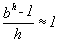, so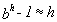and thus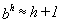. Now if we examine the natural logarithm of both sides of this approximation we have that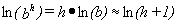. Dividing by h and using further the properties of logarithms we obtain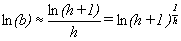. Now recall that when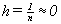, for large values of n,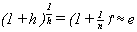.

Putting this together with the last statement we have that as h®0, ln(b)® ln(e)=1. But ln (b) is a constant. Therefore, ln (b) = 1 and it must be that b = e.

Our argument has shown that if there is a base b where the derivative of bx at 0 is 1, then that base must be the number e. By reversing the reasoning the converse statement can also be shown true. That is, we can justify the following core result:

If f(x) = ex then f '(0) = 1 and therefore f '(x) = ex = f(x) for any real number x.

 h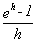1 1.718281828 0.1 1.051709181 0.01 1.005016708 0.001 1.000500167 0.0001 1.000050002 0.00001 1.000005
See Tables *** for the information on ex comparable to the work done for 2x and its derivatives.

 x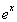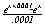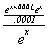-5 0.0067 0.006738 1.000005 -4 0.0183 0.0183157 1.000005 -3 0.0498 0.0497873 1.000005 -2 0.1353 0.135336 1.000005 -1 0.3679 0.3678813 1.000005 0 1 1.000005 1.000005 1 2.7183 2.7182954 1.000005 2 7.3891 7.389093 1.000005 3 20.086 20.085637 1.000005 4 54.598 54.598423 1.000005 5 148.41 148.4139 1.000005
4. What does .69315 have to do with the number 2? This is a strange coincidence which will make more sense when we delve a little more deeply into exponential functions in chapter II. If you check on your calculator you will find that ln(2) is approximately .69314718 which is very close to the value we found for the derivative of 2x at 0. In chapter ** we will see that for any base b, Dx(bx) = bx ln(b) and then we will be able to complete the list of derivative for core functions with the derivative of the logarithmic functions. Here is a summary of this discussion about simple exponential functions:
Theorem: I.F.6. (The Proportional Derivative of Exponential Functions.) If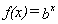where b>0 then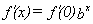. [We will show later in Chapter II that f '(0) = ln(b).] In particular if f(x) = ex, then f '(0) = 1 and therefore f '(x) = ex = f(x) for any real number x. In operator and Leibniz notations this last statement is written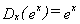and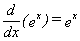.

Example. I.F.5. Find the derivative of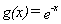.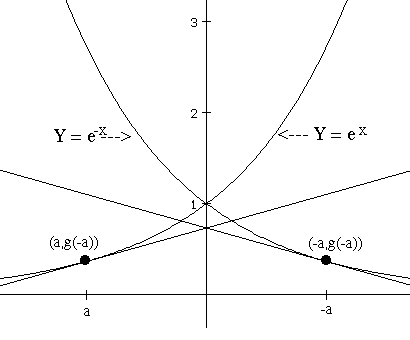Solution: We will look at this from a graphical and an algebraic viewpoint. From Figure *** as well as the simple algebra we can see that g(-a)= e-(-a)= e a.

On the graph this result is seen in the fact that the graph of g(x) is the mirror image - reflection of the graph of e x. Considering the tangent line interpretation of the derivative, we see that the tangent line for the graph of g(x) at the point (-a, g(-a)) will be the mirror image - reflection of the tangent line to the graph of e x at the point (a, e a). The slope of this line is the derivative of e x at a, namely e a. The mirror image line will have the opposite slope, so the derivative of g at -a, g '(-a) = - e a. Now think of any number x as the opposite of a number, x=-a, so a=-x. Then the last statement says that g '(x) = g '(-a) = - e a =- e -x.

Here's another way to see this result using the symmetry of the graphs and algebra: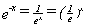so g(x) is a simple exponential function with base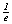. From the symmetry of the graphs of g(x) and e x, the slope of the tangent line to the graph of g at (0,1) is -1, so g '(0)= -1. See Figure *** Thus from the result we have about derivative of simple exponential functions we have that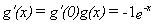.

The derivative of ln- the natural logarithm.

Here  is a brief approach to finding the derivative of the natural logarithm. Logarithmic functions with other bases may be done similarly. The logarithmic functions will be treated again in Chapter II as well as Chapter VI.So suppose f (x) = ln(x) and we want to find f '(a). We will use the difference quotient. The idea is simply to substitute y for f(x) and b for f(a). The definition of the logarithm gives us that if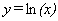then ey = x and similarly if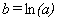then e b = a. It should make sense from your experience with logarithms that when x approaches a, y approaches b. You should draw a transformation figure for ln(x) and ex to help visualize these last statements.

Now let's consider the difference quotient,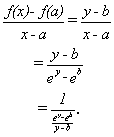.
It's again time to think about the limit, this time as y®b. The denominator is the difference quotient for the derivative of ex evaluated at b, so it approaches eb and so we see that.To summarize, we have shown that for x>0,or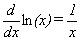.

Note: Notice how this result makes sense with the graphical interpretation. See Figure ***. When x is close to 0, the slope is quite large, while when x is large the slope of the tangent line slope seems closer to 0.

Derivatives of Other Logarithms: From your background (or the quick review box)you may recall that if we know the natural logarithm of a number x>0 and a base b>0 b¹1,then the logarithm with the base b is determined by the equation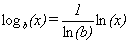. Applying the scalar multiple rule to this expression of the general logarithm function with base b we have that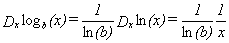and.

Thus for example,.

Exercises I.F.2. In problems 1 to 3 find the indicated derivatives.

1. Find f '(1) and f '(-1) using an estimate when appropriate:
1. f (x)= 2 x + 6x - 3
2. f (t)= 5 t + t
3. f (u)= u 20 - 4 u
4. f (z)= ez + 1/z
5. f (x)= ln(x)+ 1/x.
1. Find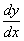when
1. y = 5 sin (x) - 3 cos (x); y = ln(x 2) + sin (x)
2. y = cos(x) - 5x - 3 ; y = 3 sin (x) + 1/ x
1. Find f '(x) and g'(x) when
1. f (x)= ex+e-x ; g (x)= ex-e-x
2. f (x)= 2ex+3e-x ; g (x)= 2ex-3e-x
3. f (x)= Aex+Be-x ; g (x)= Aex-Be-x
1. Notice in Problem 3 how the derivatives of the pairs of functions f and g are related.
1. Find a pair of functions f and g where f '(x) = g(x) and g '(x) = f(x) where f (0)=1 and g(0) = 0.
2. Find a pair of functions f and g where f '(x) = g(x) and g '(x) = f(x) where f (0)=4 and g(0) = 3.
1. Find all x where f ' (x) = 0 when
1. f(x) = sin(x)
2. f(x) = sin(x) + x
3. f(x) = cos(x)
4. f(x) = cos(x) - x
5. f(x) = sin(x) + cos(x)
1. Consider F(x) = sin(x) is a probability distribution for the random variable X on the interval [0, p/2]. Find the probability density at x=p/4, p/6, and p/3. Explain briefly why the values for X are more likely to fall closer to p/6 then to p/4 or p/3.
2. Consider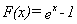is a probability distribution for the random variable X on the interval [0, ln(2)]. Find and estimate as a decimal the probability density at x=.2, .4, and .6. Explain briefly why the values for X are more likely to fall closer to .6 then to .4 or .2.
3. Consider F(x)= ln(x) as a probability distribution for the random variable X on the interval [1, e]. Find and estimate as a decimal the probability density at x= 1.5, 2, and 2.5. Explain briefly why the values for X are more likely to fall closer to 1.5 then to 2 or 2.5.
4. Prove Fact I.F.8. ii). Use this to prove Theorem I.F.9.ii).
5. Find a function P where P'(x) = f(x) for the following:

6. a. f(x) = 5 cos(x) - 3/x 2 + 2x 3/5 b. f(x) = 2 sin(x) - 3x 2 + 2x 5/7 .
7. Estimate f '(0) for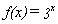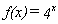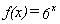and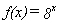. Can you see any arithmetical relations between these derivatives and the derivative of 2x at 0?
8. Look in some biology or physics book to find models that use a simple exponential function to describe a variable that can be measured experimentally. Describe the context for the model and how the model is used to either explain or predict.
9. Read some of Napier's original paper (Text in html ) developing the concept of a logarithm. Write a short paper discussing his use of a motion interpretation to explain how the logarithms would help in calculating products, etc.